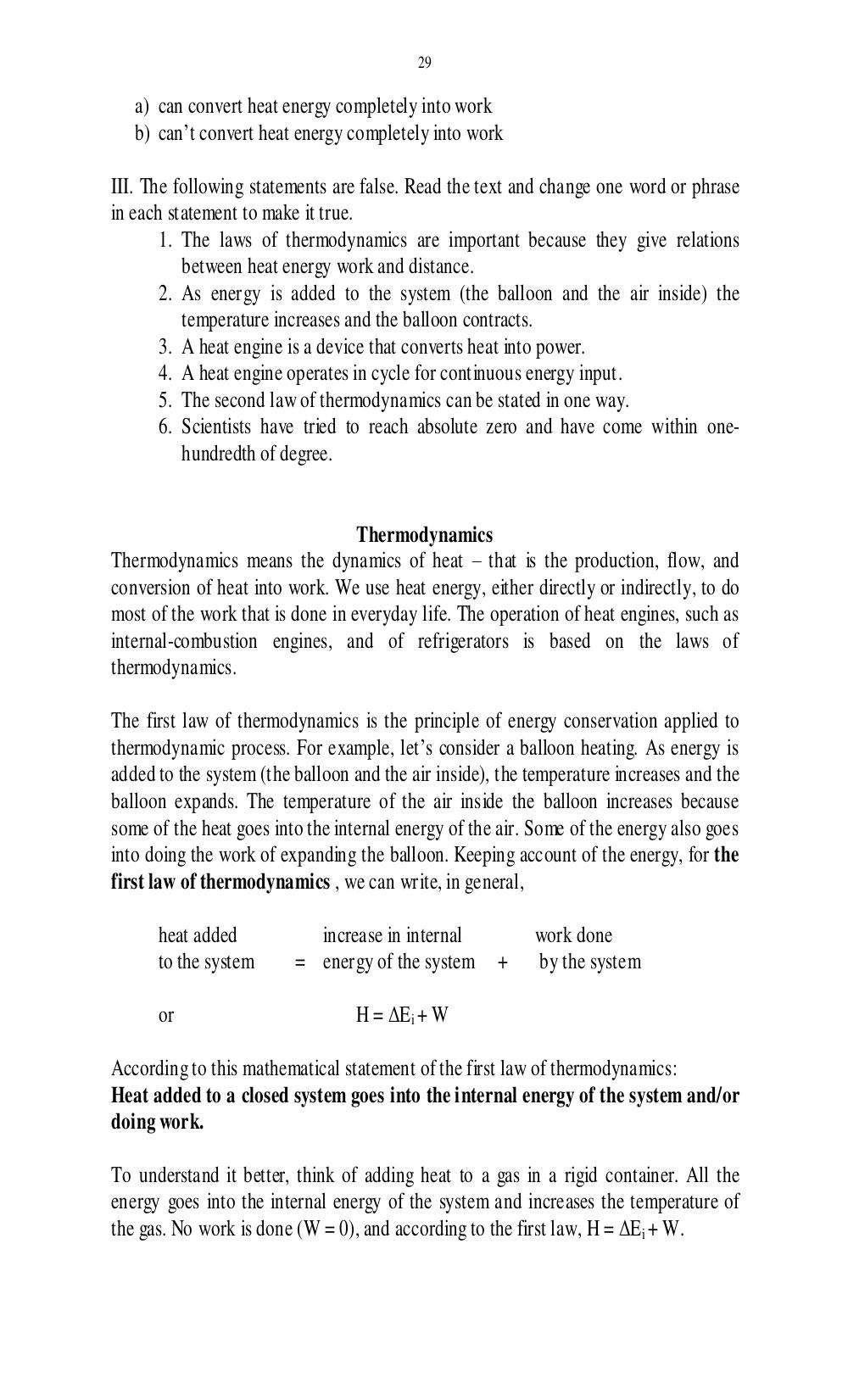# Учебно-методическое пособие по чтению специальной литературы для студентов 1 курса физического факультета. Дроздова И.В - 29 стр.

Составители:

Рубрика:

• ## Иностранный язык

29
a) can convert heat energy completely into work
b) cant convert heat energy completely into work
III. The following statements are false. Read the text and change one word or phrase
in each statement to make it true.
1. The laws of thermodynamics are important because they give relations
between heat energy work and distance.
2. As energy is added to the system (the balloon and the air inside) the
temperature increases and the balloon contracts.
3. A heat engine is a device that converts heat into power.
4. A heat engine operates in cycle for continuous energy input.
5. The second law of thermodynamics can be stated in one way.
6. Scientists have tried to reach absolute zero and have come within one-
hundredth of degree.
Thermodynamics
Thermodynamics means the dynamics of heat that is the production, flow, and
conversion of heat into work. We use heat energy, either directly or indirectly, to do
most of the work that is done in everyday life. The operation of heat engines, such as
internal-combustion engines, and of refrigerators is based on the laws of
thermodynamics.
The first law of thermodynamics is the principle of energy conservation applied to
thermodynamic process. For example, lets consider a balloon heating. As energy is
added to the system (the balloon and the air inside), the temperature increases and the
balloon expands. The temperature of the air inside the balloon increases because
some of the heat goes into the internal energy of the air. Some of the energy also goes
into doing the work of expanding the balloon. Keeping account of the energy, for the
first law of thermodynamics , we can write, in general,
heat added increase in internal work done
to the system = energy of the system + by the system
or H = Δ E
i
+ W
According to this mathematical statement of the first law of thermodynamics:
Heat added to a closed system goes into the internal energy of the system and/or
doing work.
To understand it better, think of adding heat to a gas in a rigid container. All the
energy goes into the internal energy of the system and increases the temperature of
the gas. No work is done (W = 0), and according to the first law, H = ΔE
i
+ W.29

a) can convert heat energy completely into work
b) can’t convert heat energy completely into work

III. The following statements are false. Read the text and change one word or phrase
in each statement to make it true.
1. The laws of thermodynamics are important because they give relations
between heat energy work and distance.
2. As energy is added to the system (the balloon and the air inside) the
temperature increases and the balloon contracts.
3. A heat engine is a device that converts heat into power.
4. A heat engine operates in cycle for continuous energy input.
5. The second law of thermodynamics can be stated in one way.
6. Scientists have tried to reach absolute zero and have come within one-
hundredth of degree.

Thermodynamics
Thermodynamics means the dynamics of heat – that is the production, flow, and
conversion of heat into work. We use heat energy, either directly or indirectly, to do
most of the work that is done in everyday life. The operation of heat engines, such as
internal-combustion engines, and of refrigerators is based on the laws of
thermodynamics.

The first law of thermodynamics is the principle of energy conservation applied to
thermodynamic process. For example, let’s consider a balloon heating. As energy is
added to the system (the balloon and the air inside), the temperature increases and the
balloon expands. The temperature of the air inside the balloon increases because
some of the heat goes into the internal energy of the air. Some of the energy also goes
into doing the work of expanding the balloon. Keeping account of the energy, for the
first law of thermodynamics , we can write, in general,

heat added           increase in internal           work done
to the system      = energy of the system      +    by the system

or                         H = ΔEi + W

According to this mathematical statement of the first law of thermodynamics:
Heat added to a closed system goes into the internal energy of the system and/or
doing work.

To understand it better, think of adding heat to a gas in a rigid container. All the
energy goes into the internal energy of the system and increases the temperature of
the gas. No work is done (W = 0), and according to the first law, H = ΔE i + W.